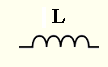# Inductor Impedance Calculator

The impedance $Z_L$ of an inductor of inductance $L$, in complex form, is given by$Z_L = j \; X_L$
where $j$ is the imaginary unit and $X_L = \omega \; L$ is the inductive reactance in Ohms $( \Omega )$
In , $Z_L$ is writtean as
$Z_L = X_L \; \angle \; 90^{\circ}$ or $Z_L = X_L \; \angle \; \dfrac{\pi}{2}$
$\omega = 2 \pi f$ is the angular frequency in radians per second (rad/s) and $f$ is the frequency in Hertz (Hz).
This calculator calculates angular frequency $\omega$, the inductive reactance $X_L$ and the impedance $Z_L$ in complex standard and polar forms.

## Use of the calculator

Enter the inductance $L$ and the frequency $f$ and press "Calculate".

 Inductance L = 20 nH μH mH H Frequency f = 1.5 GHz MHz kH Hz mHz Number of Decimal Places = 5

## Results

in complex form
in polar form with phase in degrees
in polar form with phase in radians